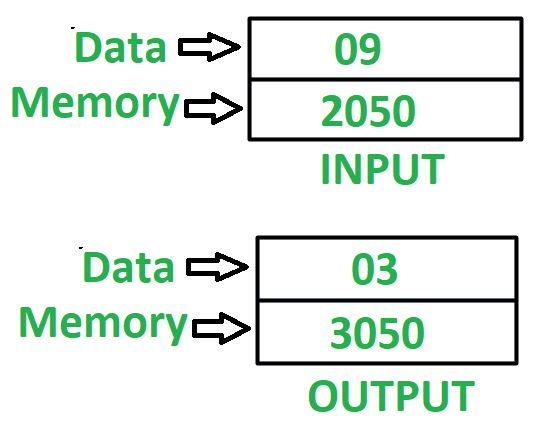# 8085 program to find square root of a number

• Last Updated : 28 Jun, 2020

Problem – Write an assembly language program in 8085 microprocessor to find square root of a number.

Example –

Attention reader! Don’t stop learning now. Get hold of all the important CS Theory concepts for SDE interviews with the CS Theory Course at a student-friendly price and become industry ready.Assumptions –
Number, whose square root we need to find is stored at memory location 2050 and store the final result in memory location 3050.

Algorithm –

1. Assign 01 to register D and E
2. Load the value, stored at memory location 2050 in accumulator A
3. Subtract value stored at accumulator A from register D
4. Check if accumulator holds 0, if true then jump to step 8
5. Increment value of register D by 2
6. Increment value of register E by 1
8. Move value stored at register E in A
9. Store the value of A in memory location 3050

Program –

2000MVI D, 01D <- 01
2002MVI E, 01E <- 01
2004LDA 2050A <- M
2007SUB DA <- A – D
2008JZ 2011Jump if ZF = 0 to memory location 2011
200BINR DD <- D + 1
200CINR DD <- D + 1
200DINR EE <- E + 1
2011MOV A, EA <- E
2012STA 3050A -> M
2015HLTEND

Explanation – Registers used A, D, E:

1. MVI D, 01 – initialize register D with 01
2. MVI E, 01 – initialize register E with 01
3. LDA 2050 – loads the content of memory location 2050 in accumulator A
4. SUB D – subtract value of D from A
5. JZ 2011 – make jump to memory location 2011 if zero flag is set
6. INR D – increments value of register D by 1. Since it is used two times, therefore value of D is incremented by 2
7. INR E – increments value of register E by 1